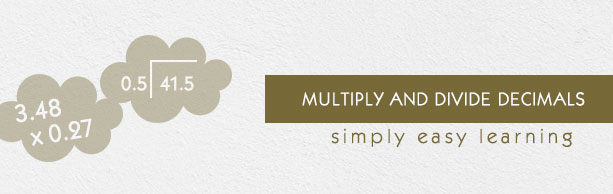# Multiply and Divide Decimals

This tutorial provides comprehensive coverage of multiplication and division of decimals based on Common Core (CCSS) and State Standards and its prerequisites. Students can navigate learning paths based on their level of readiness. Institutional users may customize the scope and sequence to meet curricular needs. This simple tutorial uses appropriate examples to help you understand multiplication and division of decimals in a general and quick way.

# Audience

This tutorial has been prepared for beginners to help them understand the basics of multiplication and division of decimals. After completing this tutorial, you will find yourself at a moderate level of expertise in multiplication and division of decimals, from where you can advance further.

# Prerequisites

Before proceeding with this tutorial, you need a basic knowledge of elementary math concepts such number sense, multiplication, division, place values of whole numbers, place value chart, definition of decimal numbers and so on.